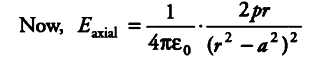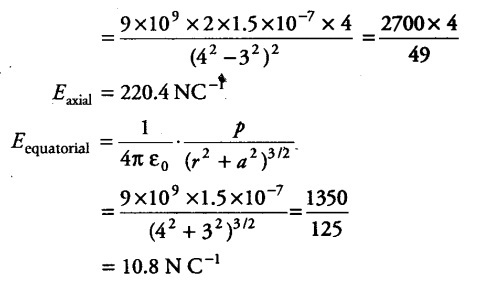# Find the electric field at a point 4 m

Two charges of+25 x \${{10}^{-9}}\$ C and - 25 x \${{10}^{-9}}\$ C are placed 6 m apart. Find the electric field at a point 4 m from the centre of the electric dipole (i) on axial line (ii) on equatorial line.

Here, q = 25 x {{10}^{-9}} C
2a = 6m , r = 4m
p = q(2a) = 25 x {{10}^{-9}} x 6 = 1.5 x {{10}^{-7}} C